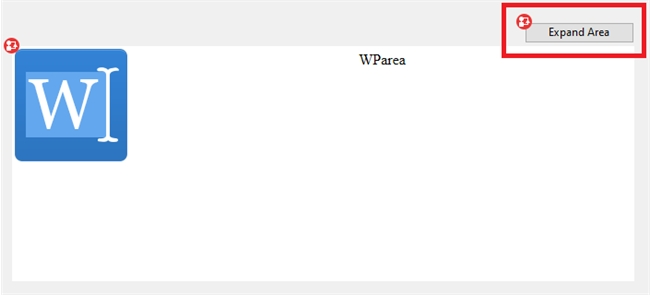KNOWLEDGE BASE
Tech Tip: 4D Write Expand Function for 4D Write Pro
PRODUCT: 4D | VERSION: 19 | PLATFORM: Mac & Win
Published On: January 25, 2023

Below is a method that can be used to simulate the expand feature of 4D Write. 4D Write had a feature in which the upper right corner of the area allowed the 4D Write Area to expand and provide a larger view of the area. This feature is replicated in 4D Write Pro by building and loading a dynamic form that only contains the 4D Write Pro area and toolbar and passing the 4D Write Pro document as the form data to maintain the data and cursor position/selection.
The feature only works for WP areas with an object variable assigned and other expressions such as a Table field will not work due to incompatibility with various commands such as OBJECT Get data source and WP selection range.

 // Method: WP_Expand// Parameters:// \$1 - Name of the 4D Write Pro Form Object to ExpandC_TEXT(\$1) // Write Pro Form Object NameIf (Count parameters=1)      C_TEXT(\$form_t)   C_OBJECT(\$form_o; \$data_o)   C_POINTER(\$dataSource_p)      // Dynamic Form Definition   \$form_t:="{\"destination\":\"detailScreen\",\"rightMargin\":20,\""   \$form_t:=\$form_t+"bottomMargin\":20,"   \$form_t:=\$form_t+"\"markerHeader\":15,\"markerBody\":200,\"markerBreak\":220,"   \$form_t:=\$form_t+"\"markerFooter\":240,\"events\":[\"onLoad\"],\"method\":\""   \$form_t:=\$form_t+Current method name+"\",\"pageFormat\":"   \$form_t:=\$form_t+"{\"paperName\":\"Letter\",\"paperWidth\":\"611pt\","   \$form_t:=\$form_t+"\"paperHeight\""+":\""+"791pt\"},\"pages\":[null,{\"objects\""   \$form_t:=\$form_t+":{\"WParea\":{\"type\":\"write\",\"top\":110,\"left\":20,"   \$form_t:=\$form_t+"\"width\":880,\"height\":590,"   \$form_t:=\$form_t+"\"sizingX\":\"grow\",\"sizingY\":"   \$form_t:=\$form_t+"\"grow\",\"dataSource\":\"Form:C1466.wparea\","   \$form_t:=\$form_t+"\"hideFocusRing\""   \$form_t:=\$form_t+":true,\"scrollbarVertical\":\"automatic\","   \$form_t:=\$form_t+"\"scrollbarHorizontal\":"   \$form_t:=\$form_t+"\"automatic\",\"dpi\":0,\"showSelection\":true,"   \$form_t:=\$form_t+"\"borderStyle\":"   \$form_t:=\$form_t+"\"none\",\"method\":\""+Current method name   \$form_t:=\$form_t+"\",\"events\":[\"onLoad\",\"onLosingFocus\",\"onGettingFocus\","   \$form_t:=\$form_t+"\"onSelectionChange\",\"onAfterEdit\"]},\"WPtoolbar\":"   \$form_t:=\$form_t+"{\"type\":\"subform\",\"top\":20,\"left\":20,\"width\":880,"   \$form_t:=\$form_t+"\"height\":90,\"sizingX\":\"grow\",\"detailForm\""   \$form_t:=\$form_t+":\"WP_Toolbar\","   \$form_t:=\$form_t+"\"focusable\":false,\"deletableInList\":false,"   \$form_t:=\$form_t+"\"doubleClickInRowAction\":\"editSubrecord\","   \$form_t:=\$form_t+"\"doubleClickInEmptyAreaAction\":\"addSubrecord\","   \$form_t:=\$form_t+"\"selectionMode\":\"multiple\","   \$form_t:=\$form_t+"\"printFrame\":\"variable\"}}}]}"      // Convert to JSON   \$form_o:=JSON Parse(\$form_t)      // Check if expression is an object variable   \$dataSource_p:=OBJECT Get data source(*; \$1)   If(Is nil pointer(\$dataSource_p)=False)     // Build Form object data with wp doc object and cursor position/selection     \$data_o:=New object     \$data_o.wparea:=\$dataSource_p->     \$data_o.position:=WP Selection range(*; \$1)          // Run Form     DIALOG(\$form_o; \$data_o)        // Update cursor position/selection when returning to calling form     WP SELECT(\$data_o.position)  Else      //Data Source is not Object Variable or not applicable      // with the OBJECT Get data source command   End If    Else    // Object Method for dynamic form      If (Form event code=On Load)     // On Load event updates the cursor position/selection to match the original "expanded" WP area     WP SELECT(Form.wparea; Form.position)   End if       // Applies Widget method for toolbar   WP UpdateWidget("WPtoolbar"; "WParea")      // Keeps track of cursor position/selection to apply to original form   If (Form event code=On Selection Change)     Form.position:=WP Selection range(*; "WParea")   End if    End if

The method is self contained and does not require any additional methods to be added or created.
An example of using the method is to apply it to a button on the same form as the target WP area to expand similar to the how the 4D Write Area behaved:The WP area's object name is \$1 and the object method of the button is:
 WP_Expand(\$1)